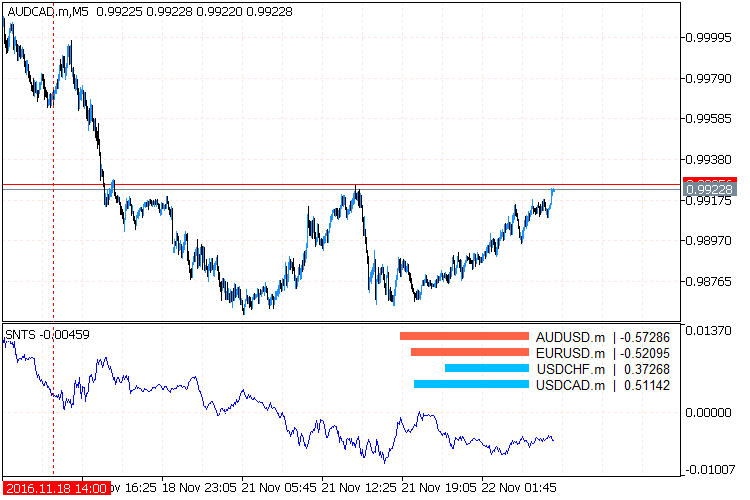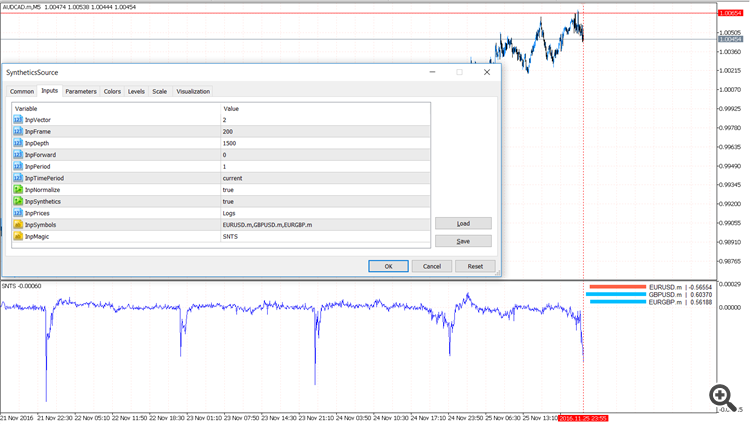PCA Synthetics – Recycle Legacy – indicator for MetaTrader 5

• Currency pairs: Any pairs, indices and metals
• Trading Time: Around the clock
• Recommended broker: icmarkets, XM
• Related Posts

Indicator for automatic selection of the coefficients for every instrument in a pseudo-stationary portfolio, which tends to equilibrium at zero.

The indicator requires the presence of the AlgLib library in the Include\Math folder of the terminal.

A Bit of Theory

Every instrument moves in its own direction, every direction is a separate dimension in a multidimensional array. Bu turning the matrix, that is, by multiplying its every element by a certain number, we try to find an axis, with the minimum distance the axis and all instruments, i.e. the least total variance. The number to multiply each element of the matrix then becomes the angle value, by which the moving instrument should be turned so that it moves in the same direction as other instruments. This angle value is the coefficient for every currency in the portfolio.

If the coefficient value is greater than 0 the currency is bought, if less than 0 — sold. Thus, it is possible to sustain the stationarity of the created synthetic by recalculating the coefficients from time to time. In addition, the PCA does not simply find the axis with the least variance for the portfolio, but several. The number of instruments in the portfolio is the number of components (vectors). Each of them is called the main component, and determines how much it affects the total change of the portfolio movement.

Possible problems

1. If the chart is not drawn, see what is printed in the Experts tab. Perhaps, there are some errors or synchronizations with other charts is in progress. If there are no messages, click through other timeframes.

2. The obtained vector values were verified with the once calculated in the R package, so the values themselves are correct. But the sign of a specific coefficient may be wrong, as the РСА does not pay attention to signs. The “-” or “+” sign can only be determined empirically, that is by trial and error.

Problem #2 with has been describes with the pictures here: http://stats.stackexchange.com/questions/34396/im-getting-jumpy-loadings-in-rollapply-pca-in-r-can-i-fix-it

Parameters

InpVector = 0; // If there are N currencies in portfolio, the movement axis number 0 = maximum variance, N – 1 = minimum
InpFrame = 300; // Floating window for calculation of coefficients, for each of InpDepth bars make InpFrame computations
InpDepth = 1000; // The total number of bars in history, for which the chart is drawn
InpForward = 500; // The bar to stop recalculating the coefficients and use the previous ones, this is OOS
InpPeriod = 1; // Smoothing for the МА, to make the chart look less twitched
InpTimePeriod = PERIOD_CURRENT; // Timeframe for the calculations
InpNormalize = true; // Normalize the prices before displaying them, to smooth the volatility gaps of USDJPY and EURGBP
InpSynthetics = true; // Draw the summary synthetic multiplied by the found coefficients or each pair individually
InpPrices = Logs; // Normalization algorithm of pairs
InpSymbols = “AUDUSD,USDCHF,USDCAD”; // Pairs for the portfolio
InpMagic = “ID” // Custom name of the indicator, to facilitate placing multiple instances on one chart without conflicts

The idea was adopted from here: https://www.mql5.com/en/code/9908• How to install MT5 indicator?
• 1. On the desktop, double-click to open the MT45 trading platform software you installed.
• 2. Click on “File” in the upper left corner – “Open Data Folder”, double-click the MQL5 folder, and double-click the Indicators folder
• 3. Copy the metrics you need to install to the Indicators folder
• 4. Open MT5, find “Navigator” in the display menu, then click “Technical Indicators” of “Navigator” and right click to refresh, you will see the indicator just copied.
• 5. In the “Technical Indicators” of “Navigator”, find the indicator file just installed, double click or click manually to the chart window